• C语言的各种字符,适用于C语言课程设计时需要用到命令行的同学
• 闲来无聊，写了一个小时候看到其他软件经常出现的字符界面。 实现了一定的通用性，字体居中等等；逻辑上没什么难度，本来是C语言写的，由于太过无聊，又改成C++了 #include&lt;iostream&gt; #include&...
闲来无聊，写了一个小时候看到其他软件经常出现的字符界面。
实现了一定的通用性，字体居中等等；逻辑上没什么难度，本来是C语言写的，由于太过无聊，又改成C++了
#include<iostream>
#include<windows.h>
#include<String.h>
#include<time.h>
#pragma comment(lib,"user32.lib")
using namespace std;

class UI{
void Pos(int x,int y);
public:
void setTime();
void surface(int x,int y,int start_x ,int start_y,WORD color);
void Information(int x,int y,string str,string author,string data,int start_x,int start_y,WORD color);
};

void UI::Pos(int x, int y){
COORD pos;
HANDLE hOutput;
pos.X = x;
pos.Y = y;
hOutput = GetStdHandle(STD_OUTPUT_HANDLE);
SetConsoleCursorPosition(hOutput, pos);
}
void UI::surface(int x,int y,int start_x =0,int start_y =0,WORD color=FOREGROUND_RED){
CONSOLE_SCREEN_BUFFER_INFO csbi;
HANDLE hConsole = GetStdHandle(STD_OUTPUT_HANDLE);
GetConsoleScreenBufferInfo(hConsole, &csbi);
start_x+=csbi.dwCursorPosition.X;
start_y+=csbi.dwCursorPosition.Y;
SetConsoleTextAttribute(GetStdHandle(STD_OUTPUT_HANDLE), color);
int sign_y = 0;
Pos(start_x,start_y+sign_y);//start
for(int i =0 ; i < x+2 ; i++){
cout << "|";
}
sign_y++;
for(int j=start_y+sign_y;j<y+start_y;j++){
Pos(start_x,j);
cout << "||";
Pos(x+start_x,j);
cout << "||";
}
sign_y+=y;
Pos(start_x,start_y+sign_y-1);
for(int i =0 ; i <= x+1 ; i++){
cout << "|";
}
sign_y++;
Pos(start_x,start_y+sign_y);
}
void UI::Information(int x,int y,string str,string author="",string data="",int start_x=0,int start_y=0,WORD color=FOREGROUND_GREEN){
CONSOLE_SCREEN_BUFFER_INFO csbi;
HANDLE hConsole = GetStdHandle(STD_OUTPUT_HANDLE);
GetConsoleScreenBufferInfo(hConsole, &csbi);
start_x+=csbi.dwCursorPosition.X;
start_y+=csbi.dwCursorPosition.Y;
SetConsoleTextAttribute(GetStdHandle(STD_OUTPUT_HANDLE), color);
int sign_y = 0;
Pos(start_x,start_y+sign_y);//start
for(int i =0 ; i < x+2 ; i++){
cout << "|";
}
sign_y++;
for(int k=0;k<3;k++){
Pos(start_x,start_y+k+sign_y);
cout << "||";
Pos(start_x+x,start_y+k+sign_y);
cout << "||";
}
sign_y+=3;
if((x-str.length())/2<0||(x-str.length())/2>x/2){
str = "错误！字符长度过长！";
}
Pos(x/2-(str.length()/2),start_y+2);
cout << str;
Pos(start_x,start_y+sign_y);
for(int i =0 ; i < x+2 ; i++){
cout << "|";
}
sign_y++;
for(int k=0;k<3;k++){
Pos(start_x,start_y+k+sign_y);
cout << "||";
Pos(start_x+x,start_y+k+sign_y);
cout << "||";
}
sign_y++;
Pos(start_x+x-6-author.length(),start_y+sign_y);//署名
cout << "作者：" << author;
sign_y++;
Pos(start_x+x-13-author.length(),start_y+sign_y);//日期
cout << "日期：" << data ;
sign_y++;
Pos(start_x,start_y+sign_y);
for(int i =0 ; i < x+2 ; i++){
cout << "|";
}
sign_y++;
Pos(start_x,start_y+sign_y);
}
void UI::setTime(){
time_t now;
struct tm *tm_now;
time(&now);
tm_now = localtime(&now);
Pos(90,20);
cout <<tm_now->tm_year+1900 << "年" << tm_now->tm_mon+1 << "月" << tm_now->tm_mday << "日" ;
Pos(92,21);
cout << tm_now->tm_hour<< "时" << tm_now->tm_min << "分" << tm_now->tm_sec << "秒";
Pos(90,30);
}
int main(){
SetConsoleTitle("测试程序");
UI ui;
ui.Information(80,30,"爱我中华","拉阿鲁","2018年7月30日");
ui.surface(80,30,0,0);
while(true){
ui.setTime();
Sleep(1000);
}
return 0;
}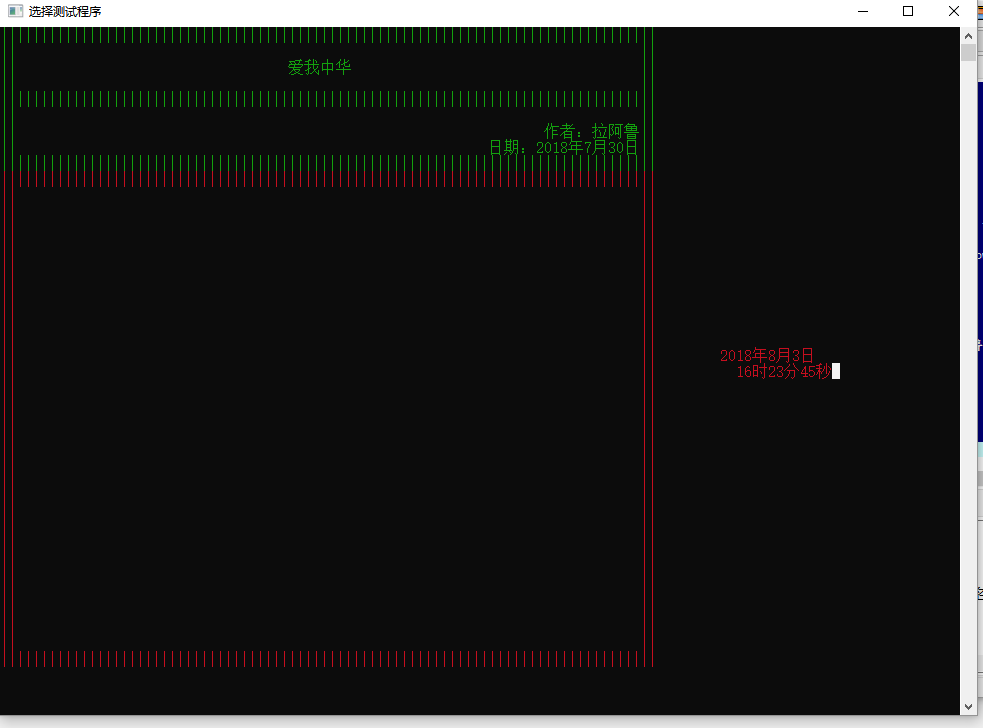展开全文• #include void aix1(x); void aix2(y); void aix3(z);... } 上面的是代码，整体思路就是，函数分别控制行间隔，输出*（爱心*够成），换行。然后每个涵数内加一层循环，数组控制循环次数。大概就是这样。

#include <stdio.h>
void aix1(x);
void aix2(y);
void aix3(z);
void aix4(j);
main(){ int a[]={20,5,16,14,12,14,16,18,20,22,24,26,27,30},
b[]={5,5,23,27,31,27,23,19,15,11,7,3,1},c[]={0,1,1,1,1,1,1,1,1,1,1,1,1,1,1},d=0,e=0,f,i=0;
kol: aix2(a[d]);
d++;
aix1(b[e]);
e++;
if(c[i]){
aix3(c[i]);
i++;}
else i++;
if(d==14);
else goto kol;
}
void aix1(x){
for(;x!=0;x--)
printf("*");
}
void aix2(y){
for(;y!=0;y--)
printf(" ");
}
void aix3(z){printf("n");
}
上面的是代码，整体思路就是，用函数分别控制行间隔，输出*（爱心用*够成），换行。然后每个涵数内加一层循环，用数组控制循环次数。大概就是这样。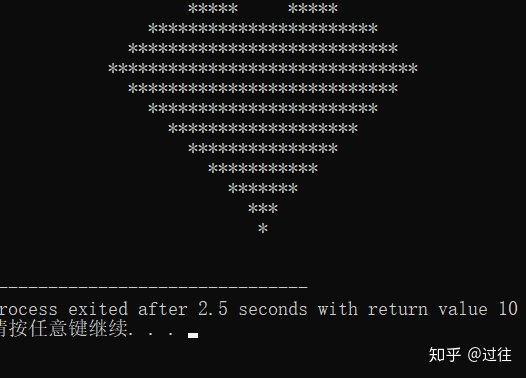展开全文• 本文借助easyx库函数（提供下载链接），很方便地用C语言实现了鼠标、按钮、进度条、坐标尺等操作，为普通C程序的友好人机交互界面提供了一种简单便捷的方案。


目录
0.引言1.素材准备2.编程2.1.创建你的界面2.2.创建按钮2.3.鼠标操作2.3.1.单击特效2.3.2.光标感应2.3.3.进度条

3.完整代码及效果

0.引言

看了CSDN上很多关于C程序图形化界面的介绍，有的代码繁琐难解，不方便调试修改；有的不够详细。本文提供的代码简单、易于移植、容易理解，望急需使用C语言制作图形化界面的朋友采纳。

对easyx尚不熟悉的朋友不需要担心，我敢打包票它只需10分钟就可以上手，而它为你节省的时间可能是3个小时甚至更多。关于easyx的简单应用请参考一篇我以前写的关于C程序可视化的博文。 →【C语言实现动画控制】←

本文的讲解是循序渐进的，读者应该重点关注每个步骤的理解，两步之间代码的变化，即可完全理解本文。
1.素材准备
easyx的下载链接如下：（本文使用的版本是2014冬至版） https://www.easyx.cn/downloads/ 注：使用easyx需要注意它兼容的编译器（下载的帮助文件会写），不同的easyx兼容的编译器不同，但总是和visual C++6兼容（和字符编码有关），本文以visual C++6编译器为例书写代码。easyx的最新英文帮助文档链接（下载2014冬至版会自带中文帮助文档）： https://docs.easyx.cn/en-us/intro如果你成功下载了easyx2014冬至版，那么解压后把头文件（easyx.h和graphic.h）和lib文件（amd64）分别放在VC文件夹默认的include文件夹和lib文件夹中。右键你的VC程序，选择打开文件所在位置，然后找到MFC文件夹，友情提供两个文件夹的位置截图。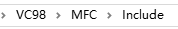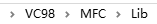建议编译的C文件以cpp后缀保存。
2.编程
2.1.创建你的界面

创建一个480×360的窗口，我们需要使用initgraph()函数，闲言少叙，让我们直接看一段代码：
#include <graphics.h>              // 引用图形库头文件
#include <conio.h>
#include <stdio.h>
#include <windows.h>				//用到了定时函数sleep()
#include <math.h>

int main()
{
int i;
short win_width,win_height;//定义窗口的宽度和高度
win_width = 480;win_height = 360;
initgraph(win_width,win_height);//初始化窗口（黑屏）
for(i=0;i<256;i+=5)
{
setbkcolor(RGB(i,i,i));//设置背景色，原来默认黑色
cleardevice();//清屏（取决于背景色）
Sleep(15);//延时15ms
}
closegraph();//关闭绘图界面
}



这段代码很容易理解，运行这段程序，就会出现逐渐明亮的屏幕。因为不断刷新背景色为

R

G

B

(

i

,

i

,

i

)

RGB(i,i,i)

。C语言中的颜色使用十六进制表示的，RGB函数可以将0~255范围内的三个整数三原色转换成这个十六进制。

cleardevice()函数用于清屏，是界面内所有元素都被清空，一般只会在初始化出现。

Sleep()是毫秒级延迟，当然界面变亮时间不一定是准确的15ms×255/5=0.765s，因为其他语句还需要执行时间。

closegraph()：关闭绘图界面。注意，如果初始化了绘图界面但没有在主函数结束前关闭它，可能会引发一些莫名其妙的错误！所以这个函数一定要有！
2.2.创建按钮

我们尝试在界面创建几个按钮，按钮需要的操作是绘制矩形和打印文字。虽然看着简单，但是里面还是有点学问，为了方便大家理解，还是先放上代码和注释。
#include <graphics.h>              // 引用图形库头文件
#include <conio.h>
#include <stdio.h>
#include <windows.h>				//用到了定时函数sleep()
#include <math.h>
int r1[]={30,20,130,60};//输入按钮的矩形参数
int r2[]={170,20,220,60};//运行按钮的矩形参数
int r3[]={260,20,310,60};//退出按钮的矩形参数
int main()
{
int i;
short win_width,win_height;//定义窗口的宽度和高度
win_width = 480;win_height = 360;
initgraph(win_width,win_height);//初始化窗口（黑屏）
for(i=0;i<256;i+=5)
{
setbkcolor(RGB(i,i,i));//设置背景色，原来默认黑色
cleardevice();//清屏（取决于背景色）
Sleep(15);//延时15ms
}
RECT R1={r1,r1,r1,r1};//矩形指针R1
RECT R2={r2,r2,r2,r2};//矩形指针R2
RECT R3={r3,r3,r3,r3};//矩形指针R3
LOGFONT f;//字体样式指针
gettextstyle(&f);					//获取字体样式
_tcscpy(f.lfFaceName,_T("宋体"));	//设置字体为宋体
f.lfQuality = ANTIALIASED_QUALITY;    // 设置输出效果为抗锯齿
settextstyle(&f);                     // 设置字体样式
settextcolor(BLACK);				//BLACK在graphic.h头文件里面被定义为黑色的颜色常量
drawtext("输入参数",&R1,DT_CENTER | DT_VCENTER | DT_SINGLELINE);//在矩形区域R1内输入文字，水平居中，垂直居中，单行显示
drawtext("运行",&R2,DT_CENTER | DT_VCENTER | DT_SINGLELINE);//在矩形区域R2内输入文字，水平居中，垂直居中，单行显示
drawtext("退出",&R3,DT_CENTER | DT_VCENTER | DT_SINGLELINE);//在矩形区域R3内输入文字，水平居中，垂直居中，单行显示
setlinecolor(BLACK);
rectangle(r1,r1,r1,r1);
rectangle(r2,r2,r2,r2);
rectangle(r3,r3,r3,r3);
system("pause");//暂停，为了显示
closegraph();
return 0;
}这里需要特别介绍的是矩形指针

p

R

e

c

t

pRect

，它使用句柄RECT定义，并且不可以中途再次赋值。之所以要设置矩形指针了为了打印字体的时候以矩形为边界自动填充。它的格式是RECT r={X1,Y1,X2,Y2}，X1和X2是矩形的左边和右边的横坐标，Y1和Y2是矩形的上边和下边的纵坐标，这一点和rectangle()绘制空心矩形函数参数排列一致。后面的DT_CENTER | DT_VCENTER | DT_SINGLELINE就是描述填充格式的常量。使用drawtext书写文字不需要再计算文字的坐标和设置大小，会方便很多。

LOGFONT是字体样式指针，通过gettextstyle()函数来获取当前的字体类型，再通过settextstyle()函数加以设置。这里只修改了字体的名称和显示质量，还可以修改斜体、下划线等属性，更详细的部分请参考帮助文档。
2.3.鼠标操作
2.3.1.单击特效

作为一个图形化界面的C程序，鼠标操作总不能少吧。在讲解程序前先别着急，简单为大家科普一下鼠标事件：

鼠标是输入设备，只要发生以下的事件，就会暂存在鼠标消息列表中，我们的操作系统就会依次响应列表中的鼠标消息事件，常用的鼠标事件如下：
WM_MOUSEMOVE——鼠标移动WM_MOUSEWHEEL——鼠标滚轮滚动WM_LBUTTONDOWN——鼠标左键按下WM_LBUTTONUP——鼠标左键弹起WM_LBUTTONDBLCLK——鼠标左键双击WM_RBUTTONDOWN——鼠标右键按下WM_RBUTTONUP——鼠标右键弹起WM_RBUTTONDBLCLK——鼠标左键双击WM_MBUTTONDOWN——鼠标中键按下WM_MBUTTONUP——鼠标中键弹起WM_MBUTTONDBLCLK——鼠标中键双击

我们只需要根据不断获取鼠标消息队列的消息并根据消息依次进行响应即可。

相信大家已经迫不及待了，那么请看下面一个简单的程序。
#include <graphics.h>              // 引用图形库头文件
#include <conio.h>
#include <stdio.h>
#include <windows.h>				//用到了定时函数sleep()
#include <math.h>
int r1[]={30,20,130,60};//输入按钮的矩形参数
int r2[]={170,20,220,60};//运行按钮的矩形参数
int r3[]={260,20,310,60};//退出按钮的矩形参数
int main()
{
int i;
short win_width,win_height;//定义窗口的宽度和高度
win_width = 480;win_height = 360;
initgraph(win_width,win_height);//初始化窗口（黑屏）
for(i=0;i<256;i+=5)
{
setbkcolor(RGB(i,i,i));//设置背景色，原来默认黑色
cleardevice();//清屏（取决于背景色）
Sleep(15);//延时15ms
}
RECT R1={r1,r1,r1,r1};//按钮1的矩形区域
RECT R2={r2,r2,r2,r2};//按钮2的矩形区域
RECT R3={r3,r3,r3,r3};//按钮2的矩形区域
LOGFONT f;
gettextstyle(&f);					//获取字体样式
_tcscpy(f.lfFaceName,_T("宋体"));	//设置字体为宋体
f.lfQuality = ANTIALIASED_QUALITY;    // 设置输出效果为抗锯齿
settextstyle(&f);                     // 设置字体样式
settextcolor(BLACK);				//BLACK在graphic.h头文件里面被定义为黑色的颜色常量
drawtext("输入参数",&R1,DT_CENTER | DT_VCENTER | DT_SINGLELINE);//在矩形区域R1内输入文字，水平居中，垂直居中，单行显示
drawtext("运行",&R2,DT_CENTER | DT_VCENTER | DT_SINGLELINE);//在矩形区域R2内输入文字，水平居中，垂直居中，单行显示
drawtext("退出",&R3,DT_CENTER | DT_VCENTER | DT_SINGLELINE);//在矩形区域R3内输入文字，水平居中，垂直居中，单行显示
setlinecolor(BLACK);
rectangle(r1,r1,r1,r1);
rectangle(r2,r2,r2,r2);
rectangle(r3,r3,r3,r3);
MOUSEMSG m;//鼠标指针
setrop2(R2_NOTXORPEN);//二元光栅——NOT(屏幕颜色 XOR 当前颜色)
while(true)
{
m = GetMouseMsg();//获取一条鼠标消息
if(m.uMsg==WM_LBUTTONDOWN)
{
for(i=0;i<=10;i++)
{
setlinecolor(RGB(25*i,25*i,25*i));//设置圆颜色
circle(m.x,m.y,2*i);
Sleep(25);//停顿2ms
circle(m.x,m.y,2*i);//抹去刚刚画的圆
}
FlushMouseMsgBuff();//清空鼠标消息缓存区
}
}
system("pause");//暂停，为了显示
closegraph();
return 0;
}每点击鼠标以下，应该可以看到鼠标点击处有一个逐渐扩大并淡出的圆（截图无法清晰，在画面的中右侧），当循环体内Sleep的视觉大于20ms后视觉效果很强。

每响应一次鼠标左键单击事件，都会调用一次清空鼠标消息缓存区的函数FlushMouseMsgBuff()，如果没有这个函数会怎么样呢？如果我们快速连续地单击鼠标左键N次，那么特效就会播放N次，如果特效播放速度比单击的速度慢，那么即使你停下来了，程序仍然会接着播放单击特效，因为你的左键单击仍然在鼠标的消息队列m.uMsg中的鼠标消息没有响应完。

这里需要解释的是一个二元光栅设置函数setrop2()，二元光栅是混合背景色和当前颜色的模式。我们这里采用的方式是同或（NOT XOR）的方式，若底色为白色（1），则当前颜色不变；若底色是黑色（0），则当前颜色反色。为什么需要采用这种方式呢？因为我们在第二次抹去原来的圆的时候不能采用白色，否则如果背景色原来就为黑（比如按钮和文字），就也会被抹成白色。而背景色与任意一个颜色同或两次都为其本身，即可起到还原背景色的效果。这里的背景色与cleardevice()前面那个背景色不同，这里的是指执行这一条绘画指令之前屏幕上的颜色。
2.3.2.光标感应

我们希望鼠标移到按钮上时按钮会有所变化，移开按钮时又会回到原样。这里我们采用一种简单的填充颜色的方法，就是按钮变色。我们需要解决一个问题就是按钮变色了但是按钮的文字不能被覆盖，那么我们还是需要使用到二元光栅。只是我们这次的模式改成了同或。

为了方便起见，存放三个按钮的数组我们合并为了一个二维数组，在鼠标事件中更容易使用和分配任务。
#include <graphics.h>              // 引用图形库头文件
#include <conio.h>
#include <stdio.h>
#include <windows.h>				//用到了定时函数sleep()
#include <math.h>
int r={{30,20,130,60},{170,20,220,60},{260,20,310,60}};//三个按钮的二维数组

int button_judge(int x,int y)
{
if(x>r && x<r && y>r && y<r)return 1;
if(x>r && x<r && y>r && y<r)return 2;
if(x>r && x<r && y>r && y<r)return 3;
return 0;
}
int main()
{
int i,event=0;
short win_width,win_height;//定义窗口的宽度和高度
win_width = 480;win_height = 360;
initgraph(win_width,win_height);//初始化窗口（黑屏）
for(i=0;i<256;i+=5)
{
setbkcolor(RGB(i,i,i));//设置背景色，原来默认黑色
cleardevice();//清屏（取决于背景色）
Sleep(15);//延时15ms
}
RECT R1={r,r,r,r};
RECT R2={r,r,r,r};
RECT R3={r,r,r,r};
LOGFONT f;
gettextstyle(&f);					//获取字体样式
_tcscpy(f.lfFaceName,_T("宋体"));	//设置字体为宋体
f.lfQuality = ANTIALIASED_QUALITY;    // 设置输出效果为抗锯齿
settextstyle(&f);                     // 设置字体样式
settextcolor(BLACK);				//BLACK在graphic.h头文件里面被定义为黑色的颜色常量
drawtext("输入参数",&R1,DT_CENTER | DT_VCENTER | DT_SINGLELINE);//在矩形区域R1内输入文字，水平居中，垂直居中，单行显示
drawtext("运行",&R2,DT_CENTER | DT_VCENTER | DT_SINGLELINE);//在矩形区域R2内输入文字，水平居中，垂直居中，单行显示
drawtext("退出",&R3,DT_CENTER | DT_VCENTER | DT_SINGLELINE);//在矩形区域R3内输入文字，水平居中，垂直居中，单行显示
setlinecolor(BLACK);
rectangle(r,r,r,r);
rectangle(r,r,r,r);
rectangle(r,r,r,r);
MOUSEMSG m;//鼠标指针

while(true)
{
m = GetMouseMsg();//获取一条鼠标消息

switch(m.uMsg)
{
case WM_MOUSEMOVE:
setrop2(R2_XORPEN);
setlinecolor(LIGHTCYAN);//线条颜色为亮青色
setlinestyle(PS_SOLID, 3);//设置画线样式为实现，10磅
setfillcolor(WHITE);//填充颜色为白色
if(button_judge(m.x,m.y)!=0)
{
if(event != button_judge(m.x,m.y))
{
event = button_judge(m.x,m.y);//记录这一次触发的按钮
fillrectangle(r[event-1],r[event-1],r[event-1],r[event-1]);//有框填充矩形（X1,Y1,X2,Y2）
}
}
else
{
if(event != 0)//上次触发的按钮未被修正为原来的颜色
{
fillrectangle(r[event-1],r[event-1],r[event-1],r[event-1]);//两次同或为原来颜色
event = 0;
}
}
break;
case WM_LBUTTONDOWN:
setrop2(R2_NOTXORPEN);//二元光栅——NOT(屏幕颜色 XOR 当前颜色)
for(i=0;i<=10;i++)
{
setlinecolor(RGB(25*i,25*i,25*i));//设置圆颜色
circle(m.x,m.y,2*i);
Sleep(30);//停顿30ms
circle(m.x,m.y,2*i);//抹去刚刚画的圆
}
break;
FlushMouseMsgBuff();//清空鼠标消息缓存区
}
}
system("pause");//暂停，为了显示
return 0;
}这里我们运用了两次设置二元光栅的函数setrop2，在鼠标的移动条件内(case MOUSEMOVE)，我们使用的是屏幕颜色和当前颜色异或，这是为什么呢？因为fillrectangle()函数是画一个有框填充矩形，我们让这个有框填充矩形和原按钮的一样大。由于线条的颜色为亮青色，填充颜色为白色（1），白色的填充颜色和屏幕颜色异或，取的是屏幕颜色的反色。按钮的边框是黑色（0），它与亮青色异或，则会保留原来的亮青色。

与同或一样，异或两次等于没有执行操作，所以可以还原到原屏幕画布的颜色。
2.3.3.进度条

既然涉及到进度条了，那么就应该涉及到正式程序了，这里我不想涉及太多的专业知识影响大家理解，但又不能让程序运行得太快以至于看不出进度条的变化，所以我设计了一个简单的弹性球轨迹作图程序。

假设球半径为R，初始高度为

h

0

h_0

，初速度为0（自由落体），非弹性碰撞时能量损失率为

α

\alpha

。计算部分子函数如下：
int simulation()
{
float dt = 0.01;//仿真间隔10ms
long int N = (long int)(sim_t/dt);//迭代次数
float *h=(float*)calloc(N,sizeof(float));//高度
float *v=(float*)calloc(N,sizeof(float));//速度（竖直方向）
long int i;//迭代变量
for(i=1;i<N;i++)
{
if(h[i-1]>R)//未发生碰撞
{
v[i]=v[i-1]-9.8*dt;//速度计算
}
else//发生碰撞，动能损失alpha，速度损失alpha的开方
{
v[i]=-sqrt(alpha)*v[i-1];
}
}
free(h);
free(v);//释放内存
return 0;
}


当然，我们还需要绘图网格，定义绘图网格的函数如下：
void init_figure()
{
int i;
setrop2(R2_COPYPEN);//当前颜色
setlinecolor(BLACK);
setlinestyle(PS_SOLID);//实线
rectangle(30,100,420,330);//外框线
setlinestyle(PS_DOT);//点线
for(i=30+39;i<420;i+=39)
{
line(i,100,i,330);//竖直辅助线
}
for(i=100+23;i<330;i+=23)
{
line(30,i,420,i);//水平辅助线
}
}


注意，我们使用了rectangle()空心矩形函数绘制网格外框架，使用了line函数依次画出了辅助线。

我们现在的目标就是将h的坐标转换到网格上去，绘制出球心的轨迹，这样似乎并不复杂，只需要对simulation()函数稍加修改即可。
int simulation()
{
float dt = 0.01;//仿真间隔10ms
float dy = 230/h0;//单位纵坐标
long int N = (long int)(sim_t/dt);//迭代次数
float *h=(float*)calloc(N,sizeof(float));//高度
float *v=(float*)calloc(N,sizeof(float));//速度（竖直方向）
long int i;//迭代变量
float process_duty;//进度
init_figure();//初始化图像网格
setrop2(R2_COPYPEN);//当前颜色
//计算步骤
h=h0;v=0;
for(i=1;i<N;i++)
{
if(h[i-1]>R)//未发生碰撞
{
v[i]=v[i-1]-9.8*dt;//速度计算
}
else//发生碰撞，动能损失alpha，速度损失alpha的开方
{
v[i]=-sqrt(alpha)*v[i-1];
}
h[i]=h[i-1]+v[i]*dt;//高度计算
process_duty = (i+1)/(float)(N);
putpixel(30+(int)(process_duty*390),330-(int)(h[i]*dy),RED);//画点putpixel(X,Y,color*)
Sleep(dt*1000);//延时
}
free(h);
free(v);
return 0;
}


这里的新函数putpixel（X,Y,color*）是画像素点的函数，适合刻画不连续或不规则的移动轨迹。

现在我们只剩下了刻画进度条的函数了，进度条的刷新很明显是应该放在for循环里面的，那么我们采用什么进度条的格式呢？进度条可以有圆形、扇形、长条连续型、长条不连续型等多种，我们这里采用的是环形进度条，将进度数字显示在环中心。请看以下的对simulation()函数改进的代码：
//仿真运行
int simulation()
{
char t;//百分值的字符
char *out_text;//带百分号的百分字符
float dt = 0.01;//仿真间隔10ms
float dy = 230/h0;//单位纵坐标
long int N = (long int)(sim_t/dt);//迭代次数
float *h=(float*)calloc(N,sizeof(float));//高度
float *v=(float*)calloc(N,sizeof(float));//速度（竖直方向）
long int i;//迭代变量
float process_duty;//进度
RECT r={370,35,400,65};//百分值显示区域的矩形指针
init_figure();//初始化图像网格
setrop2(R2_COPYPEN);//当前颜色
setfillcolor(WHITE);
setlinecolor(WHITE);
fillrectangle(354,19,411,81);//覆盖原进度条区域
setlinestyle(PS_NULL);//无线条
setbkmode(TRANSPARENT);//设置文字填充背景为透明
//计算步骤
h=h0;v=0;
BeginBatchDraw();//开始缓存区
for(i=1;i<N;i++)
{
if(h[i-1]>R)//未发生碰撞
{
v[i]=v[i-1]-9.8*dt;//速度计算
}
else//发生碰撞，动能损失alpha，速度损失alpha的开方
{
v[i]=-sqrt(alpha)*v[i-1];
}
setfillcolor(WHITE);
setlinecolor(WHITE);
fillrectangle(354,19,416,81);//覆盖原进度条区域
h[i]=h[i-1]+v[i]*dt;//高度计算
process_duty = (i+1)/(float)(N);
setlinestyle(PS_SOLID);
putpixel(30+(int)(process_duty*390),330-(int)(h[i]*dy),RED);
setfillcolor(BLUE);
setlinestyle(PS_NULL);
fillpie(355,20,415,80,0,process_duty*2*PI);
setfillcolor(WHITE);
fillcircle(385,50,20);
sprintf(t,"%d",(int)(process_duty*100.0));//整型转换为字符串
out_text = strcat(t,"%");//添加一个百分号
drawtext(out_text,&r,DT_CENTER | DT_VCENTER | DT_SINGLELINE);
Sleep(dt*1000);
FlushBatchDraw();//刷新缓存区
}
EndBatchDraw();//结束缓存区
free(h);
free(v);
return 0;
}


这里我们需要多加载一个头文件<string.h>。

首先需要计算进度条的坐标，把环形进度条区域用白色矩形刷新掉，环形进度条需要一个扇形和圆形的组合，扇形的角度是0~360°。这里我们用到了fillpie(X1,Y1,X2,Y2,start_angle,end_angle)，前四个参数为椭圆扇形的外接矩形坐标，后两个参数分别为起始角和终止角（弧度制）。每过一次迭代都重新计算终止角（起始角始终为0），即可起到扇形角度逐渐增长的效果，再用一个白色填充圆覆盖中心部分即可变成环形进度条。

FlushBatchDraw()函数是刷新缓存区的函数，与BeginBatchDraw()和EndBatchDraw()一起使用，如果我们绘图之后不想立即显示，而想批量绘图最后一起刷新画板，用缓存区的方法再合适不过了。
3.完整代码及效果
#include <graphics.h>              // 引用图形库头文件
#include <conio.h>
#include <stdio.h>
#include <windows.h>				//用到了定时函数sleep()
#include <math.h>
#include <string.h>
#define PI 3.1416
int r={{30,20,130,60},{170,20,220,60},{260,20,310,60}};//三个按钮的二维数组
float alpha,R,h0,sim_t;//碰撞时的能量损失率，球的半径、初始高度、仿真时间
//按钮判断函数
int button_judge(int x,int y)
{
if(x>r && x<r && y>r && y<r)return 1;
if(x>r && x<r && y>r && y<r)return 2;
if(x>r && x<r && y>r && y<r)return 3;
return 0;
}
//初始化图像
void init_figure()
{
int i;
setrop2(R2_COPYPEN);//当前颜色
setlinecolor(BLACK);
setlinestyle(PS_SOLID);//实线
rectangle(30,100,420,330);//外框线
setlinestyle(PS_DOT);//点线
for(i=30+39;i<420;i+=39)
{
line(i,100,i,330);//竖直辅助线
}
for(i=100+23;i<330;i+=23)
{
line(30,i,420,i);//水平辅助线
}
}
//仿真运行
int simulation()
{
char t;//百分值的字符
char *out_text;
float dt = 0.01;//仿真间隔10ms
float dy = 230/h0;//单位纵坐标
long int N = (long int)(sim_t/dt);//迭代次数
float *h=(float*)calloc(N,sizeof(float));//高度
float *v=(float*)calloc(N,sizeof(float));//速度（竖直方向）
long int i;//迭代变量
float process_duty;//进度
RECT r={370,35,400,65};//百分值显示区域的矩形指针
init_figure();//初始化图像网格
setrop2(R2_COPYPEN);//当前颜色
setfillcolor(WHITE);
setlinecolor(WHITE);
fillrectangle(354,19,411,81);//覆盖原进度条区域
setlinestyle(PS_NULL);//无线条
setbkmode(TRANSPARENT);//设置文字填充背景为透明
//计算步骤
h=h0;v=0;
BeginBatchDraw();//开始缓存区
for(i=1;i<N;i++)
{
if(h[i-1]>R)//未发生碰撞
{
v[i]=v[i-1]-9.8*dt;//速度计算
}
else//发生碰撞，动能损失alpha，速度损失alpha的开方
{
v[i]=-sqrt(alpha)*v[i-1];
}
setfillcolor(WHITE);
setlinecolor(WHITE);
fillrectangle(354,19,416,81);//覆盖原进度条区域
h[i]=h[i-1]+v[i]*dt;//高度计算
process_duty = (i+1)/(float)(N);
setlinestyle(PS_SOLID);
putpixel(30+(int)(process_duty*390),330-(int)(h[i]*dy),RED);
setfillcolor(BLUE);
setlinestyle(PS_NULL);
fillpie(355,20,415,80,0,process_duty*2*PI);
setfillcolor(WHITE);
fillcircle(385,50,20);
sprintf(t,"%d",(int)(process_duty*100.0));//整型转换为字符串
out_text = strcat(t,"%");//添加一个百分号
drawtext(out_text,&r,DT_CENTER | DT_VCENTER | DT_SINGLELINE);
Sleep(dt*1000);
FlushBatchDraw();//刷新缓存区
}
EndBatchDraw();//结束缓存区
free(h);
free(v);
return 0;
}

int main()
{
int i,event=0;
char s;//输入字符串变量
short win_width,win_height;//定义窗口的宽度和高度
win_width = 480;win_height = 360;
initgraph(win_width,win_height);//初始化窗口（黑屏）
for(i=0;i<256;i+=5)
{
setbkcolor(RGB(i,i,i));//设置背景色，原来默认黑色
cleardevice();//清屏（取决于背景色）
Sleep(30);//延时30ms
}
RECT R1={r,r,r,r};
RECT R2={r,r,r,r};
RECT R3={r,r,r,r};
LOGFONT f;//字体样式指针
gettextstyle(&f);					//获取字体样式
_tcscpy(f.lfFaceName,_T("宋体"));	//设置字体为宋体
f.lfQuality = ANTIALIASED_QUALITY;    // 设置输出效果为抗锯齿
settextstyle(&f);                     // 设置字体样式
settextcolor(BLACK);				//BLACK在graphic.h头文件里面被定义为黑色的颜色常量
drawtext("输入参数",&R1,DT_CENTER | DT_VCENTER | DT_SINGLELINE);//在矩形区域R1内输入文字，水平居中，垂直居中，单行显示
drawtext("运行",&R2,DT_CENTER | DT_VCENTER | DT_SINGLELINE);//在矩形区域R2内输入文字，水平居中，垂直居中，单行显示
drawtext("退出",&R3,DT_CENTER | DT_VCENTER | DT_SINGLELINE);//在矩形区域R3内输入文字，水平居中，垂直居中，单行显示
setlinecolor(BLACK);
rectangle(r,r,r,r);
rectangle(r,r,r,r);
rectangle(r,r,r,r);
MOUSEMSG m;//鼠标指针

while(true)
{
m = GetMouseMsg();//获取一条鼠标消息

switch(m.uMsg)
{
case WM_MOUSEMOVE:
setrop2(R2_XORPEN);
setlinecolor(LIGHTCYAN);//线条颜色为亮青色
setlinestyle(PS_SOLID, 3);//设置画线样式为实现，10磅
setfillcolor(WHITE);//填充颜色为白色
if(button_judge(m.x,m.y)!=0)
{
if(event != button_judge(m.x,m.y))
{
event = button_judge(m.x,m.y);//记录这一次触发的按钮
fillrectangle(r[event-1],r[event-1],r[event-1],r[event-1]);//有框填充矩形（X1,Y1,X2,Y2）
}
}
else
{
if(event!=0)//上次触发的按钮未被修正为原来的颜色
{
fillrectangle(r[event-1],r[event-1],r[event-1],r[event-1]);//两次同或为原来颜色
event = 0;
}
}
break;
case WM_LBUTTONDOWN:
setrop2(R2_NOTXORPEN);//二元光栅——NOT(屏幕颜色 XOR 当前颜色)
for(i=0;i<=10;i++)
{
setlinecolor(RGB(25*i,25*i,25*i));//设置圆颜色
circle(m.x,m.y,2*i);
Sleep(20);//停顿30ms
circle(m.x,m.y,2*i);//抹去刚刚画的圆
}
//按照按钮判断左键单击后的操作
switch(button_judge(m.x,m.y))
{
//复原按钮原型
case 1:
InputBox(s,30,"请输入碰撞时的能量损失率、球的半径、初始高度、仿真时间");
sscanf(s,"%f%f%f%f",&alpha,&R,&h0,&sim_t);//将输入字符串依次扫描到全局变量里面
FlushMouseMsgBuffer();//单击事件后清空鼠标消息
break;
case 2:
simulation();//仿真运行
FlushMouseMsgBuffer();//单击事件后清空鼠标消息
break;
case 3:
closegraph();//关闭绘图环境
exit(0);//正常退出
default:
FlushMouseMsgBuffer();//单击事件后清空鼠标消息
//printf("\r\n(%d,%d)",m.x,m.y);//打印鼠标坐标，方便调试时确定区域
break;
}
break;
}
}
return 0;
}希望本文对您有帮助，谢谢阅读。
展开全文控制台 可视化
• c语言字符界面快译通源码,请注意源码的文本格式是gbk的喔.dict.txt 是词库.dict1.c 是源码 .可以gcc 编译。当然在g++也可以编译
• 3.字符界面：屏幕根据“按需刷新”原则，每次只是刷新有变化的区域。总体上个人感觉还不错啦，哈哈！莫喷…… 4.代码设计：状态加定时器驱动，个人感觉可以满足大多数单线程小游戏的开发。 环境：code::blocks + ...
• 用C语言写的走迷宫小游戏，简单的字符界面操作，使用自然分岔法生成迷宫地图，本人大一新生，代码也许比较粗糙，大神勿笑。
• 跟之前的贪吃蛇一样，都是C写的，字符界面的小游戏，没有调用到任何三方图形库。 都是调用到了之前封装好的几个资源: JR_timer JR_Random JR_KeyBoard JR_Cursor 环境：code::blocks + MinGW PS:有的朋友VS...
• 这是用C语言写的计算器。有界面哦！欢迎大家下载！
• ## 【C语言开发】登陆界面

万次阅读 多人点赞 2018-10-11 11:43:21
system("title 登录界面"); //设置屏幕为白底红字 system("color f4"); //设置窗口大小为80×40 system("mode con cols=80 lines=40"); //显示系统时间 showtime(); //绘制心形图案 paintheart(); /...
要求：
1.显示系统时间
2.绘制系统图案
3.输入用户名和密码，并保存到data.txt文件中

//define.h

#ifndef _DELINE_H
#define _DELINE_H

#include <stdio.h>
#include <time.h>
#include <string.h>
#include <stdlib.h>

//显示系统时间
void showtime();
//绘制心形
void paintheart();
//输入用户名
int scanfuser();
//输入密码

#endif
//showtime.cpp

#include "define.h"

void showtime()
{
time_t timep;
char s;
time(&timep);
strcpy(s,ctime(&timep));
printf("%s", s);
}
//paintheart.cpp

#include "define.h"

void paintheart()
{
//心形函数
for (float y = 1.5f; y > -1.5f; y -= 0.1f)
{
for (float x = -1.5f; x < 1.5f; x += 0.05f)
{
float a = x * x + y * y - 1;
putchar(a * a * a - x * x * y * y * y <= 0.0f ? '*' : ' ');
}
putchar('\n');
}
}
//scanfuser.cpp

#include "define.h"

int scanfuser()
{
//定义用户名数组
char user;

//打印文字
printf("用户名：");

//输入用户名并保存到user数组中
scanf("%s",user);

//printf("%s",user);

//将用户名信息保存在data.txt文件中
FILE *fpWrite=fopen("data.txt","a+");

//成功创建文件时
if(fpWrite)
{
//不为空时逐个字符保存
for(int i=0;user[i]!='\0';i++)
{
fprintf(fpWrite,"%c",user[i]);
}

//关闭文件
fclose(fpWrite);

//释放指针
fpWrite=NULL;
}
return 0;
}

//scanfpassword.cpp

#include "define.h"

{
//声明密码数组

//打印文字
printf("密码：");

//输入密码并保存到数组中

//将用户名信息保存在data.txt文件中
FILE *fpWrite=fopen("data.txt","a+");

//成功创建文件时
if(fpWrite)
{
//不为空时逐个字符保存
{
}

//关闭文件
fclose(fpWrite);

//释放指针
fpWrite=NULL;
}
return 0;
}
//main.cpp

#include "define.h"

int main()
{
//更改标题
system("title 登录界面");

//设置屏幕为白底红字
system("color f4");

//设置窗口大小为80×40
system("mode con cols=80 lines=40");

//显示系统时间
showtime();

//绘制心形图案
paintheart();

//换行居中
printf("\n                     ");

//输入用户名
scanfuser();

//换行居中
printf("\n                     ");

//输入密码

return 0;
}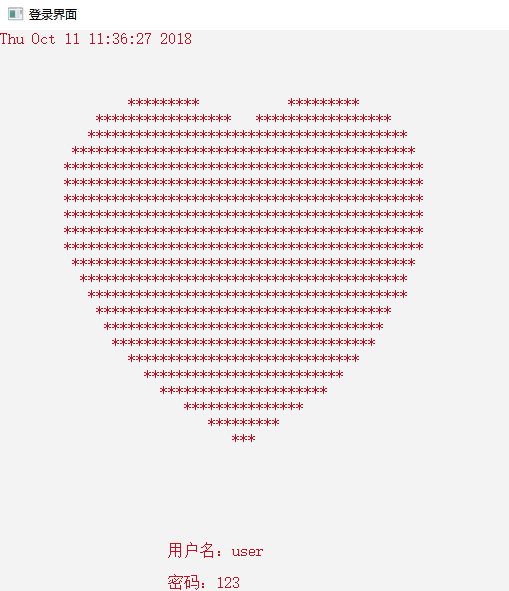展开全文• 时磊忖呎 时磊忖呎 经典C语言程序设计100例 1.数字排列 37.对10个数进行排序 70.计算一个字符串长度 2.奖金分配问题 38.求3*3矩阵对角线元素之和 71.编写输入/输岀函数 3.已知条件求解整数 72.创建链表 4.输入日期...
• 主要面向想做出图形界面C语言初学者 C语言初学者开始都是在黑白控制台上开发一些小程序，实现一些小功能，因为基础的C语言可视化效果比较差，所展示的都是黑白的字符和数字，比较单调乏味。一些大学的计算机类专业...
• c语言字符界面跳舞机小游戏c语言字符界面跳舞机小游戏c语言字符界面跳舞机小游戏
• ## C语言控制台窗口图形界面编程

万次阅读 多人点赞 2017-03-26 13:05:25
用C语言编写控制台的窗口界面首先要获取当前标准输入和标准输出设备的句柄。通过调用函数GetStdHandle可以获取当前标准输入以及输出设备的句柄。函数原型为： [cpp]   view plain   copy ...
• C语言字符串的比较，如何判定字符串相同？ C语言小知识： 注意点: 因为不确定字符串的长度，所以使用while循环， 再比较到字符串的串尾 #include<stdio.h> int main(void) { char arr1[]=...
• ## c语言实现字符串

千次阅读 2016-08-21 01:16:58
串的基本概念串 ( 字符串 ) :是零个或多个字符组成的有限序列。 记作: S=”a1a_1a2a_2a3a_3a4a_4…” ,其中 S 是串名, aia_i (1 ≦ i ≦ n) 是 单个,可以是字母、数字或其它字符。 串值:双引号括起来的字符序列是串...
• * 参数: buf: 字符类型指针，屏幕输入的字符的存放地 * 返回值: 1：从屏幕输入的字符串存放成功 * 0：从屏幕输入的字符串存放失败 */ int input_string(char *buf) { int i = 0...
• 字符界面解决乱码问题 dos界面 如何解决字符乱码问题
• char x={{'7','8','9','+'},//将图形界面信息二维数组表示 {'4','5','6','-'}, {'1','2','3','*'}, {'.','0','=','/'}}; MOUSEMSG m; RECT r={1,1,400,100};//RECT 参数名：...c
• * Version 1.0 * * 某水果店有如下商品 苹果 桃子 香蕉 哈密瓜 葡萄 店老板要设计一程序能根据客户反馈的信息要什么水果多少斤来出货 程序界面参考如下图所示的那样 * 内容的分析大致如下 好根据我们学过的知识设计...
• 显示器基本都有字符模式和图形显示模式，用C语言实现色彩斑斓的图形显示。 1、为了能使用C语言，我们需要设置栈空间。C语言函数体中的变量就是使用栈管理的，默认情况下C语言编译后生成的代码是能在该操作系统下...
• 我的第一个程序 代码如下： #include void main() { printf("我的第一个程序：hello ... //输出c的值 } printf是C语言库函数，功能是向标准输出设备输出一个字符串 printf(“hello world\n”);//\n的意思是回车换行
• 跟之前的贪吃蛇和俄罗斯方块一样，都是C写的，字符界面的小游戏，没有调用到任何三方图形库。 功能介绍： 一、正常游戏 二、手动编辑地图（编辑中可以选择随机生成） 三、保存地图（可以保存自己编辑的地图，也...
• C语言写的字符界面迷宫小游戏（原创）- release版，欢迎下载试玩交流！
• 上表中各功能对应的C语言知识 成绩查询、更改、排序输出—>链表的排序与查找与更改 学生的增删—>链表的增删更改与文件读写 账号的增删改查—>结构体数组的增删改查与文件读写 成绩、账号申诉与登录记录—>...
• ## 停车场管理系统 课程设计C语言

万次阅读 多人点赞 2019-06-28 16:53:28
停车场管理系统课程设计 数据结构 C语言 设计内容： 设有一个可以停放n（n>=5）辆汽车的狭长停车场,它只有一个大门可以供车辆进出。车辆按到达停车场时间的早晚依次从停车场最里面向大门口处停放(最先到达的第一...停车场管理系统 数据结构
• ## C语言字符串操作总结大全(超详细)

万次阅读 多人点赞 2019-04-02 08:17:03
1）字符串操作 strcpy(p, p1) 复制字符串 strncpy(p, p1, n) 复制指定长度字符串 strcat(p, p1) 附加字符串 strncat(p, p1, n) 附加指定长度字符串 strlen§ 取字符串长度 strcmp(p, p1) 比较字符串 strcasecmp忽略...
• 这里写自定义目录标题欢迎使用Markdown编辑器新的改变功能快捷键合理的创建标题，有助于目录的生成如何改变文本的样式插入链接与图片如何插入一段漂亮的代码片生成一个适合你的列表创建一个表格设定内容居中、居左、......

# c语言用字符设计界面c语言 订阅